``````library(tidyverse)
library(lubridate)

data %>%
filter(created_at >= '2020-07-01',
created_at < '2021-01-01',
is.na(refund_state)) %>%
select(member_no, union_no, created_at) %>%
mutate(created_at = date(created_at)) %>%
group_by(member_no, union_no) %>%
slice_max(created_at, n = 1) %>%
ungroup() %>%
select(-union_no) %>%
distinct() %>%
group_by(member_no) %>%
arrange(created_at) %>%
mutate(
n_days = n(),
tier = case_when(
n_days == 1 ~ "1d",
n_days == 2 ~ "2d",
n_days == 3 ~ "3d",
n_days  > 3 ~ "3d+"),
previous = lag(created_at),
dd = interval(previous, created_at) %/% days(1))
``````

• `filter` 筛选必要的行
• `select` 筛选必要的列
• `mutate` 更改日期时间为仅日期（间隔日，不需要理会时间点）
• `group_by` 按用户和单号进行分组
• `filter` 同一单选最大日期（发现同一单有时间不一致的情况）
• `ungroup` 取消分组
• `select` 丢掉单号列
• `distinct` 针对剩下的用户id和下单日期，去重
• `group_by` 再次按用户id分组
• `arrange` 按日期在分组内排序
• `mutate` 计算活跃（购买）天数并按天数分层
• `lag` 找出当前日期的上一个日期
• `interval` 计算两个日期之间的间隔

## 转换思路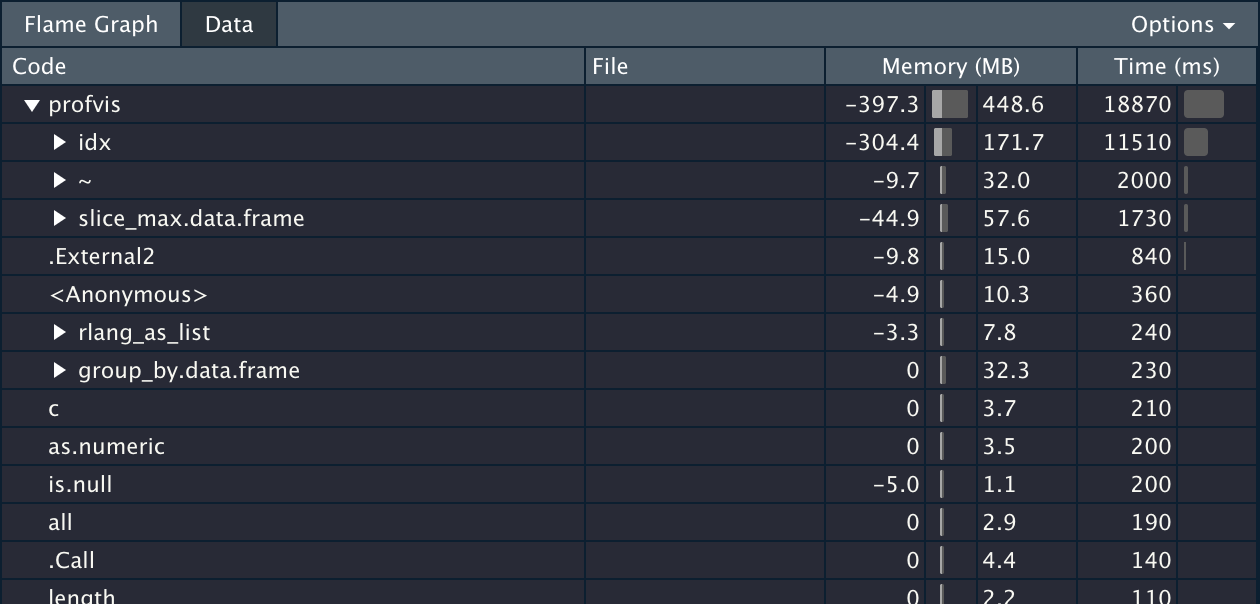### slice_ 和时区

``````data %>%
filter(created_at >= '2020-07-01',
created_at < '2021-01-01',
is.na(refund_state)) %>%
select(member_no, created_at) %>%
mutate(created_at = date(created_at)) %>%
distinct()
# ...
``````

``````TZ="UTC"
RETICULATE_PYTHON="/usr/local/bin/python3"
R_MAX_VSIZE=16Gb

http_proxy=http://127.0.0.1:1091/
https_proxy=http://127.0.0.1:1091/
``````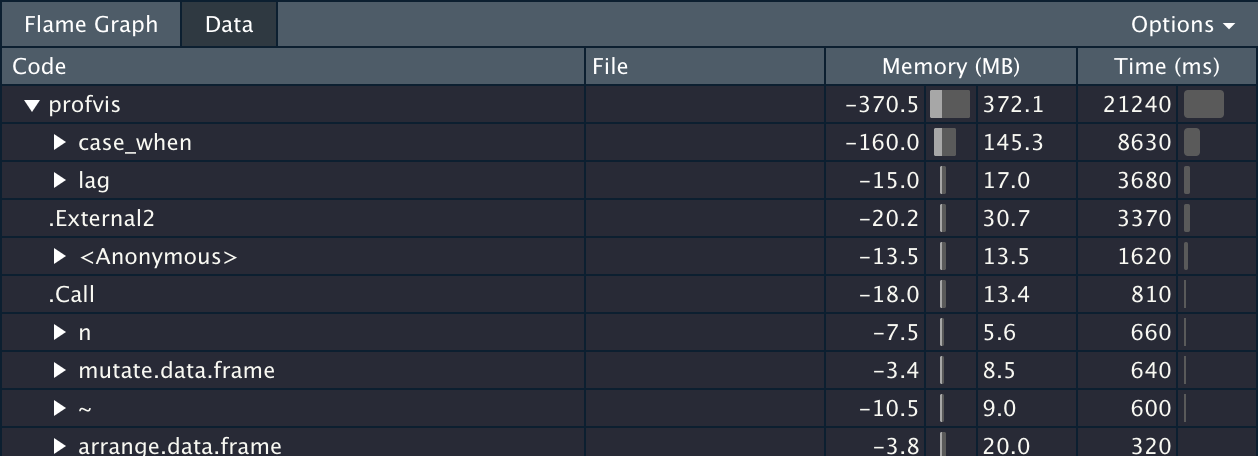`case_when``lag` 都很慢，加起来十几秒，不过，这十几秒也让最终 boss 浮出了水面：`interval` 。这里其实有点奇怪，单拿出来跑， `interval(df\$a, df\$b) %/% days(1)` 是很快的，应该是嵌套到 `mutate` 内部之后，失去了 vectorized 特性，变成了 row-wise 的循环。改用 base R 提供的 lagged differences 算法之后，就直接光速了：

``````data %>%
filter(created_at >= '2020-07-01',
created_at < '2021-01-01',
is.na(refund_state)) %>%
select(member_no, created_at) %>%
mutate(created_at = date(created_at)) %>%
distinct() %>%
group_by(member_no) %>%
arrange(created_at) %>%
mutate(
n_days = n(),
tier = case_when(
n_days == 1 ~ "1d",
n_days == 2 ~ "2d",
n_days == 3 ~ "3d",
n_days > 3 ~ "3d+"
),
dd = c(0, diff(created_at)))

``````## 更换工具

data.table 是 R 宇宙当中以性能见长的数据处理库，它的语法抽象而简洁，几乎一句话就可以概括：`DT[i, j, by]`。只是一旦 chain 到一起，（至少对我来说）就有点绕毛线了，因为它的 chain 是这样的：

``````# data.table 原生 chain
dt[][
][
][
]

# 当然也可以使用 magrittr 的 pipe
# 但内容一多，括号里面的内容就不好分割了
dt %>%
.[] %>%
.[] %>%
.[]
``````

tidyverse 语法虽然看似罗嗦，但是对比之下，在 base、tidy、data.table 这三大宇宙当中，一点点语法冗余，换来更好的排版和阅读便利，还是非常值得的。为了二者兼得，tidyverse 还在 dplyr 的基础上，提供了一个桥接方案 dtplyr，桥接的后端就是 data.table。它会在运行之前，先将 dplyr 语法转义为 data.table 语法，以期实现「写的舒服」「跑的还快」的双重目的。这个方案在之前的一些小测试中，我并没发现有大的性能差异，最近重建了 R 环境，较为仔细地完成了 openblas 和 libomp 的编译支持，正好一试。

``````dt %>% # <----
lazy_dt() %>% # <----
filter(created_at >= '2020-07-01',
created_at < '2021-01-01',
is.na(refund_state)) %>%
select(member_no, created_at) %>%
mutate(created_at = date(created_at)) %>%
distinct() %>%
group_by(member_no) %>%
arrange(created_at) %>%
mutate(
n_days = n(),
tier = case_when(
n_days == 1 ~ "1d",
n_days == 2 ~ "2d",
n_days == 3 ~ "3d",
n_days > 3 ~ "3d+"
),
dd = c(0, diff(created_at))
) %>%
as.data.table() # <----
``````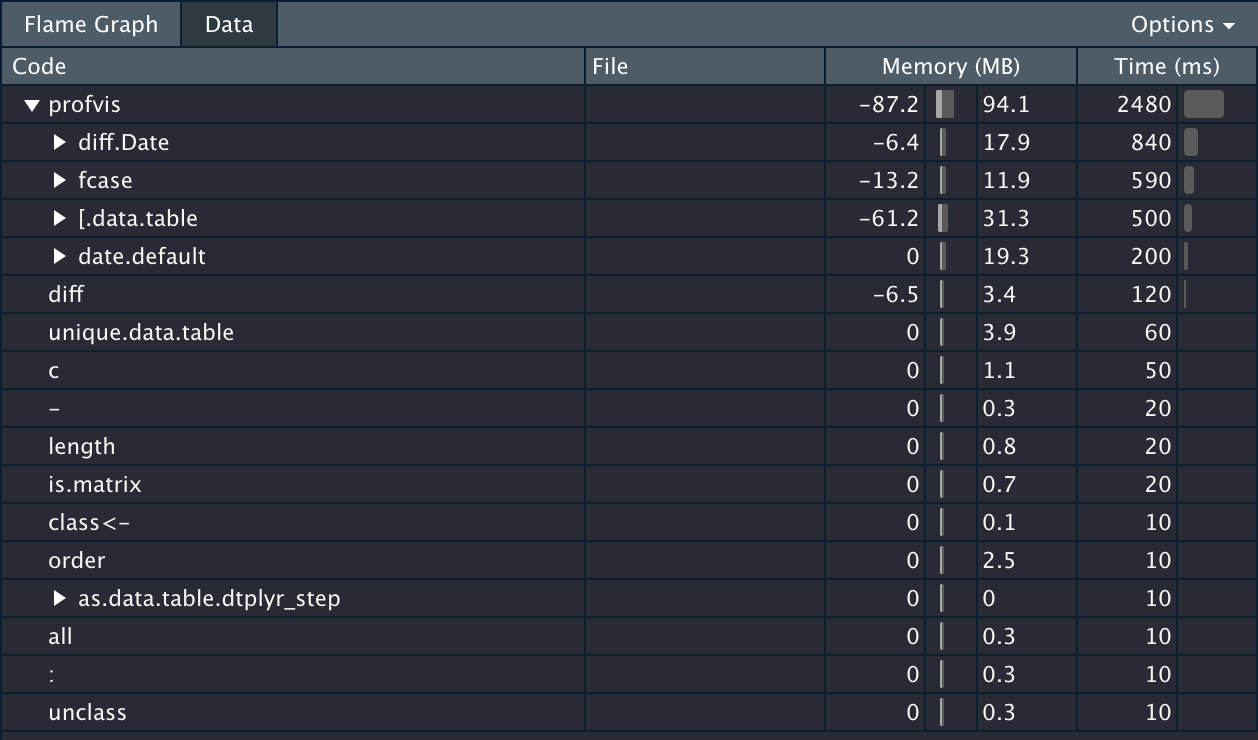``````# system.time({...})

user  system elapsed
2.915   0.037   2.098
``````

``````unique(`_DT2`[created_at >= "2020-07-01" & created_at < "2021-01-01" &
is.na(refund_state), .(member_no, created_at)][, `:=`(created_at = date(created_at))])[order(created_at)][,
`:=`(c("n_days", "tier", "dd"), {
n_days <- .N
tier <- fcase(n_days == 1, "1d", n_days == 2, "2d", n_days ==
3, "3d", n_days > 3, "3d+")
dd <- c(0, diff(created_at))
.(n_days, tier, dd)
}), by = .(member_no)]
``````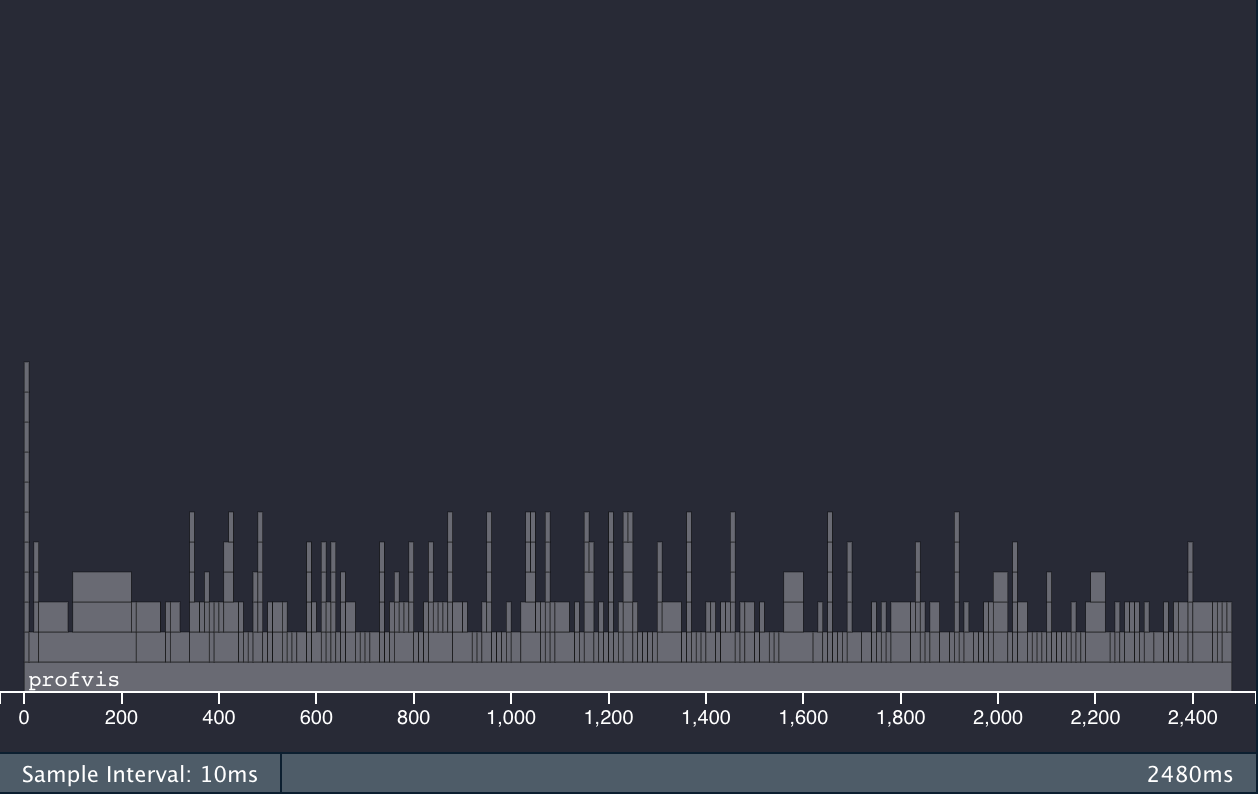dtplyr + data.table 火焰图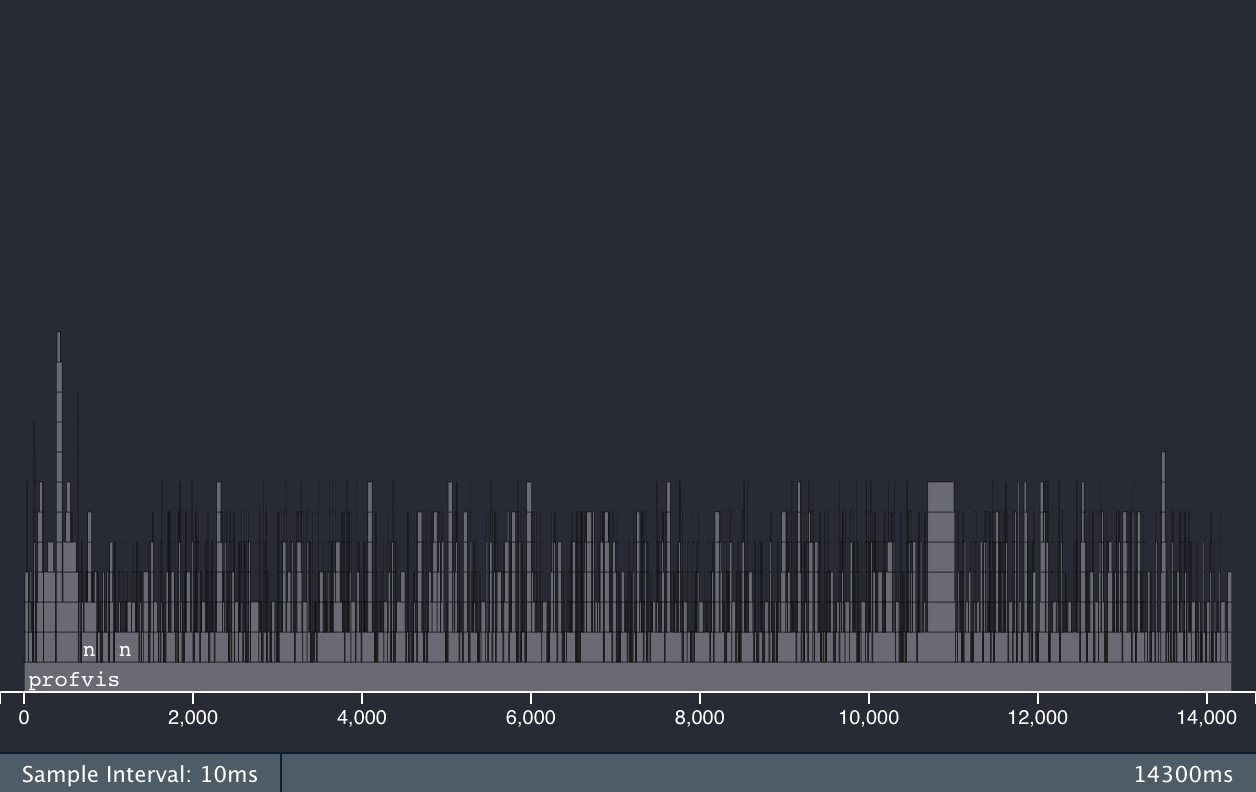dplyr 火焰图

## 小结

``````Unit: seconds
expr       min        lq      mean    median        uq      max neval
dp 18.792854 19.282197 19.793766 19.602105 20.323187 20.96849     5
dt  2.163451  2.220804  2.235009  2.234266  2.277006  2.27952     5

# 注释掉 case_when 和 diff 两处运算之后的对比
Unit: milliseconds
expr       min        lq      mean    median       uq       max neval
dp 1874.7782 1932.8641 2036.7339 2041.8302 2060.804 2273.3928     5
dt  134.3752  138.8937  155.6884  141.1073  142.066  221.9998     5

``````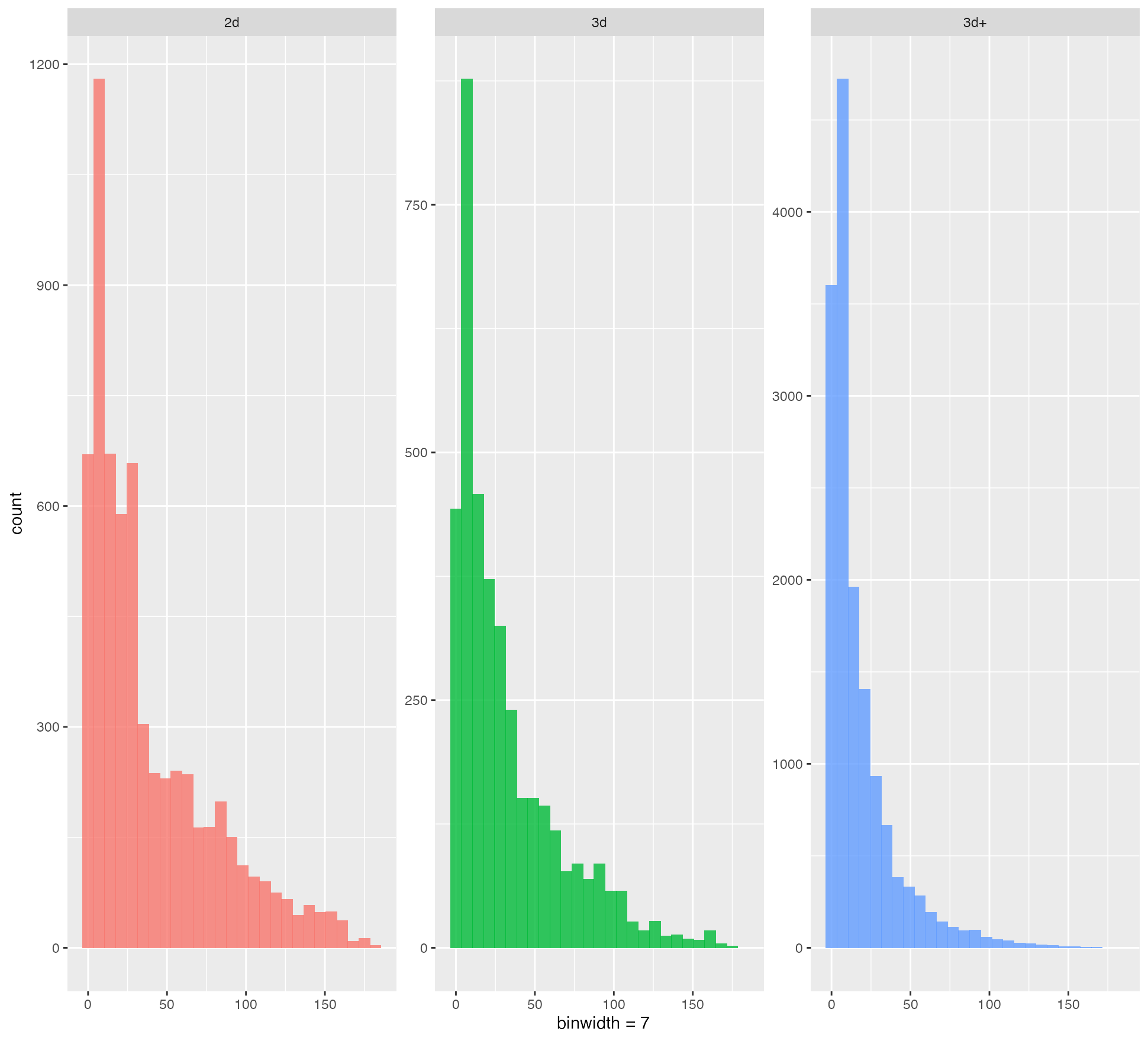样例结果绘图